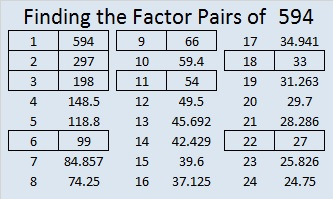# 594 and Level 2

It is as easy as 1-2-3 to remember this number fact from Stetson.edu:

(1^5) + (2^9) + (3^4) = 594

594 is the sum of the ten prime numbers from 41 to 79.

594 is even so it is divisible by 2.

5 + 9 + 4 = 18 so 594 is divisible by both 3 and 9 (and by 6 and 18 because 594 is even)

5 – 9 + 4 = 0 so 594 is divisible by 11.Print the puzzles or type the solution on this excel file: 12 Factors 2015-08-24

—————————————————————————————————

• 594 is a composite number.
• Prime factorization: 594 = 2 x 3 x 3 x 3 x 11, which can be written 594 = 2 x (3^3) x 11
• The exponents in the prime factorization are 1, 3, and 1. Adding one to each and multiplying we get (1 + 1)(3 + 1)(1 + 1) = 2 x 4 x 2 = 16. Therefore 594 has exactly 16 factors.
• Factors of 594: 1, 2, 3, 6, 9, 11, 18, 22, 27, 33, 54, 66, 99, 198, 297, 594
• Factor pairs: 594 = 1 x 594, 2 x 297, 3 x 198, 6 x 99, 9 x 66, 11 x 54, 18 x 33, or 22 x 27
• Taking the factor pair with the largest square number factor, we get √594 = (√9)(√66) = 3√66 ≈ 24.372115—————————————————————————————————